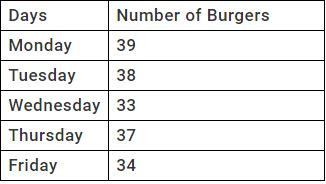Courses

# Test: Numbers - 2

## 10 Questions MCQ Test Mathematics for Class 3: NCERT | Test: Numbers - 2

Description
This mock test of Test: Numbers - 2 for Class 3 helps you for every Class 3 entrance exam. This contains 10 Multiple Choice Questions for Class 3 Test: Numbers - 2 (mcq) to study with solutions a complete question bank. The solved questions answers in this Test: Numbers - 2 quiz give you a good mix of easy questions and tough questions. Class 3 students definitely take this Test: Numbers - 2 exercise for a better result in the exam. You can find other Test: Numbers - 2 extra questions, long questions & short questions for Class 3 on EduRev as well by searching above.
QUESTION: 1

Solution:
QUESTION: 2

Solution:
QUESTION: 3

### How many odd numbers are there in between 20 and 33?

Solution:
QUESTION: 4

How many prime numbers are there in between 9 and 30?

Solution:
QUESTION: 5

On adding the least prime number to the predecessor of a number, we get 24. Find the number.

Solution:

let number be X

Least prime number is 2

according to given question 2+x=24

×=24-2

×=22

hence number is 22

QUESTION: 6

To get the successor of 17, which number should be added to 17?

Solution:
QUESTION: 7

The difference between the face values of a two digit number is 7, the number can be ________.

Solution:
QUESTION: 8

A chef recalled how many burgers he had made during the past five days.On which day did the chef make the fewest burgers?

Solution:

From the table, we cansee that on Wednesday he made only 33 burgers which is less than the number of burgers he made on all other days.

QUESTION: 9

Fill in the blank to make the following statement 29_________ 27

Solution:
QUESTION: 10

Round 694 to nearest ten,

Solution: